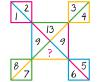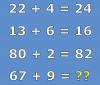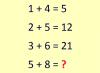BRAIN TEASERSBrain Teasers User Profile

# Eduardo Moreira

rank
526
points
3
See full ranking list
short ranking list
 524 leslie looman 3 525 Dolores Smoljanovic 3 526 Eduardo Moreira 3 527 Jelena Radin 3 528 Darko Nesovic 2
 MATH PUZZLE: Can you replace... MATH PUZZLE: Can you replace the question mark with a number?Mathematical Puzzle: IF 22+4... Mathematical Puzzle: IF 22+4=24, 13+6=16 and 80+2=82 THEN 67+9=?Calculate 5+8 If 1+4=5, 2+5=12 and 3+6=21 then 5+8=?